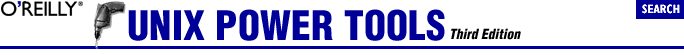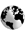home | O'Reilly's CD bookshelfs | FreeBSD | Linux | Cisco | Cisco Exam## 22.7. lensort: Sort Lines by Length

```% deroff -w report | uniq -d | lensort
a
an
...
deoxyribonucleic```

Once I used it to sort a list of pathnames:

find Section 9.1

```% find adir -type f -print | lensort
...

The script uses awk (Section 20.10) to print each line's length, followed by the original line. Next, sort sorts the lengths numerically (Section 22.5). Then sed (Section 34.1) strips off the lengths and the spaces and prints the lines:Go to http://examples.oreilly.com/upt3 for more information on: lensort

```#! /bin/sh
awk 'BEGIN { FS=RS }
{ print length, \$0 }' \$* |
# Sort the lines numerically
sort +0n -1 |
# Remove the length and the space and print each line
sed 's/^[0-9][0-9]* //'```

(Some awks require a semicolon after the first curly bracket -- that is, { FS=RS };.)

Of course, you can also tackle this problem with Perl:

```\$ perl -lne '\$l{\$_}=length;END{for(sort{\$l{\$a}<=>\$l{\$b}}keys %l){print}}' \
filename```
```#!/usr/bin/perl

my %lines;
while(my \$curr_line = <STDIN>){
chomp \$curr_line;
\$lines{\$curr_line} = length \$curr_line;
}

for my \$line (sort{ \$lines{\$a} <=> \$lines{\$b} } keys %lines){
print \$line, "\n";
}```

--JP and JJ